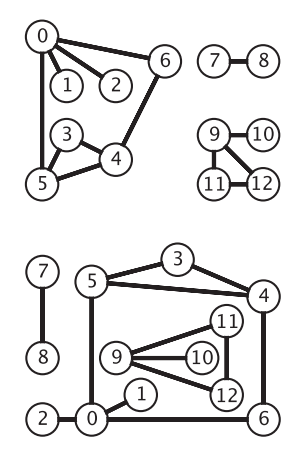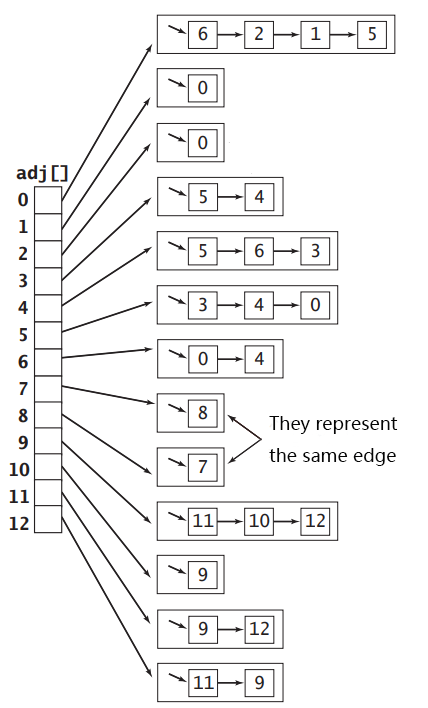# How to implement undirected graphs in Java

## Basic concepts

### Definition of a graph

A graph is a binary consisting of a set of points V={vi}V={vi} and a set E={ek}E={ek} of unordered pairs of elements in VV, denoted G=(V,E)G=(V,E), elements vivi in VV are called vertices and elements ekek in EE are called edges.

For two points u,vu,v in VV, if the edge (u,v)(u,v) belongs to EE, then the two points u,vu,v are said to be adjacent and u,vu,v is called the endpoint of the edge (u,v)(u,v).

We can denote the number of edges in the graph GG by m(G)=|E|m(G)=|E| and the number of vertices in the graph GG by n(G)=|V|n(G)=|V|.

### Definition of an undirected graph

For any edge (vi,vj)(vi,vj) in EE, if the edge (vi,vj)(vi,vj) has unordered endpoints, then it is an undirected edge, and the graph GG is then called an undirected graph. An undirected graph is the simplest graph model, and the following figure shows the same undirected graph, with vertices represented using circles and edges as lines between vertices, without arrows.## API for undirected graphs

For an undirected graph, we care about the number of vertices of the graph, the number of edges, the adjacent vertices of each vertex and the add operation of the edges, so the interface is shown below.

``````package com.javaisland.graph;

/**
* undirected graph
*/
public interface Graph {
/**
* return the number of vertices in the graph
*/
int V();

/**
* Returns the number of edges in the graph
*/
int E();

/**
* Add an edge to the graph
* @param v vertex v
* @param w vertex w
*/

/**
* @param v vertex v
*/

}
``````

## Implementation of undirected graph

Representing graphs with matrices is often convenient for studying the properties and applications of graphs. There are various matrix representations for various graphs, such as the power matrix and the adjacency matrix, and here we are only concerned with the adjacency matrix.

We can use a two-dimensional Boolean array `A` to implement the adjacency matrix when `A[i][j] = true` indicating that vertices `i` and `j` are adjacent.

For a graph GG with nn vertices, the adjacency matrix consumes a space of size n2n2 boolean values, which is very wasteful for sparse graphs and can be astronomical when the number of vertices is large. Also, when the graph is special, with self-loops and parallel edges, the adjacency matrix representation is powerless.

### Arrays of edges

For undirected graphs, we can implement a class `Edge` with only two instance variables to store the two vertices uu and vv, and then just keep all `Edges` inside an array. This has a big problem, that is, when getting all adjacent vertices of vertex vv, you have to traverse the whole array to get them, the time complexity is O(|E|)O(|E|), and since getting adjacent vertices is a very common operation, this representation is not very good.

If we represent a vertex as an integer taking values in the range 0∼|V|-10∼|V|-1, then we can represent each vertex by the index of an array of length |V||V|, and then set each array element as a chain table with the other vertices adjacent to the vertex represented by the index mounted on it. The undirected graph shown above can be represented by the array of adjacency tables shown in the following figure.The code to implement an undirected graph using adjacency tables is shown below. Since each link in the adjacency table array holds the vertices adjacent to the vertices, adding edges to the graph requires adding nodes to both links in the array as follows.

``````package com.javaisland.graph;

/**
* An undirected graph implemented using adjacency table
*/
public class LinkGraph implements Graph {

private final int V;

private int E;

this.V = V;
for (int i = 0; i < V; i++) {
}
}

@Override
public int V() {
return V;
}

@Override
public int E() {
return E;
}

@Override
public void addEdge(int v, int w) {
E++;
}

@Override
}

}
``````

The stack code used here is shown below. The implementation of the stack is not the focus of this article, so it is not explained too much here:

``````package com.javaisland.collection.stack;

import java.util.EmptyStackException;
Iterator; import java.util;

/**
* Stack implemented using a linked table
*/

private int N;

private Node first;

public void push(T item) {
first = new Node(item, first);
N++;
}

public T pop() throws EmptyStackException {
if (N == 0) {
throw new EmptyStackException();
}
T item = first.item;
first = first.next;
N --;
return item;
}

public int size() {
return N;
}

public boolean isEmpty() {
return N == 0;
}

public Iterator<T> iterator() {
return new ReverseIterator();
}

private class Node {

T item;

Node next;

public Node() {
}

public Node(T item, Node next) {
this.item = item;
this.next = next;
}
}

private class ReverseIterator implements Iterator<T> {

private Node node = first;

@Override
public boolean hasNext() {
return node ! = null;
}

@Override
public T next() {
T item = node.item;
node = node.next;
return item;
}

@Override
public void remove() {
}
}

}
``````

## Traversal of undirected graphs

Given the following graph, how do I find the path from each vertex to vertex 0? Or simply, given vertices 0 and 4, how do you determine if you can reach vertex 4 if you start at vertex 0? This requires two types of graph traversal: depth-first search and breadth-first search.

Before presenting these two traversal methods, the API that needs to be implemented to solve the above problem is given.

``````package com.javaisland.graph;

public interface Search {

/**
* whether the starting point s and vertex v are connected
* @param v vertex v
* @return whether or not to connect
*/
boolean connected(int v);

/**
* returns the number of vertices connected to vertex s (including s)
*/
int count();

/**
* whether there is a path from start s to vertex v
* @param v vertex v
* @return whether there is a path
*/
boolean hasPathTo(int v);

/**
* The path from start s to vertex v, or null if it doesn't exist
* @param v vertex v
* @return path
*/
Iterable<Integer> pathTo(int v);

}
``````

The idea of depth-first search is similar to the prior-order traversal of a tree. We start at vertex 0 and add its neighboring vertices 2, 1, and 5 to the stack. Then pop the vertex 2 at the top of the stack and add its adjacent vertices 0, 1, 3, 4 to the stack, but writing this you will find a problem: vertices 0 and 1 are already on the stack, if you add them to the stack, then the stack will never become empty. So we need to maintain an array `boolean[] marked`, and when we add a vertex `i` to the stack, we set `marked[i]` to `true`, so that the next time we want to add a vertex `i` to the stack, we have to check if `marked[i]` is `true`, and if it’s `true`, we don’t have to add it again. to the stack. Repeating the popping of the node at the top of the stack and the stacking of the nodes adjacent to the node until the stack is empty, we finish traversing all the vertices reachable by vertex 0.

To keep track of the path from each vertex to vertex 0, we also need an array `int[] edgeTo`. Whenever we visit vertex `u` and press one of its neighboring vertices `i` onto the stack, we set `edgeTo[i]` to `u`, which means that to get from vertex `i` to vertex 0, we need to backtrack to vertex `u` and then get the next vertex to backtrack to from vertex `edgeTo[u]` until we find vertex 0.

``````package com.javaisland.graph;

import com.javaisland.collection.stack;

public class DepthFirstSearch implements Search {

private boolean[] marked;

private int[] edgeTo;

private Graph graph;

private int s;

private int N;

public DepthFirstSearch(Graph graph, int s) {
this.graph = graph;
this.s = s;
marked = new boolean[graph.V()];
edgeTo = new int[graph.V()];
dfs();
}

/**
* Recursive implementation of depth-first search
*
* @param v vertex v
*/
private void dfs(int v) {
marked[v] = true;
N++;
for (int i : graph.adj(v)) {
if (!marked[i]) {
edgeTo[i] = v;
dfs(i);
}
}

}

/**

* Stacked implementation of depth-first search

*/
private void dfs() {

vertexes.push(s);

marked[s] = true;

while (!vertexes.isEmpty()) {
Integer v = vertexes.pop();
N++;
for (Integer i : graph.adj(v)) {
if (!marked[i]) {
edgeTo[i] = v;
marked[i] = true;
vertexes.push(i);
}
}
}

}

@Override
public boolean connected(int v) {
return marked[v];
}

@Override
public int count() {
return N;
}

@Override
public boolean hasPathTo(int v) {
return connected(v);
}

@Override
public Iterable<Integer> pathTo(int v) {
if (!hasPathTo(v)) return null;
int vertex = v;
while (vertex ! = s) {
path.push(vertex);
vertex = edgeTo[vertex];
}
path.push(s);
return path;
}

}
``````

The idea of breadth-first search is similar to a hierarchical traversal of a tree. Unlike depth-first search, which starts from vertex 0, breadth-first search processes all vertices 2, 1, and 5 adjacent to vertex 0 before proceeding to the vertices adjacent to vertex 2, 1, and 5. This search process is the process of expanding further and further out in a circle, so it can be used to get the shortest path from vertex 0 to other nodes. Breadth-first search can be achieved by simply replacing the heap in depth-first search with a queue: the

``````package com.javaisland.graph;

public class BreadthFirstSearch implements Search {

private boolean[] marked;

private int[] edgeTo;

private Graph graph;

private int s;

private int N;

public BreadthFirstSearch(Graph graph, int s) {
this.graph = graph;
this.s = s;
marked = new boolean[graph.V()];
edgeTo = new int[graph.V()];
bfs();
}

private void bfs() {

marked[s] = true;

queue.enqueue(s);

while (!queue.isEmpty()) {
int v = queue.dequeue();
N++;
for (Integer i : graph.adj(v)) {
if (!marked[i]) {
edgeTo[i] = v;
marked[i] = true;
queue.enqueue(i);
}
}
}

}

}
``````

The implementation code for the queue is as follows.

``````package com.javaisland.collection.queue;

import java.util.EmptyStackException;

private int N;

private Node first;

private Node last;

public void enqueue(T item) {
Node node = new Node(item, null);
if (++N == 1) {
first = node;
} else {
last.next = node;

}
last = node;

}

public T dequeue() throws EmptyStackException {
if (N == 0) {
throw new EmptyStackException();
}
T item = first.item;
first = first.next;
if (--N == 0) {
last = null;
}
return item;
}

public int size() {
return N;
}

public boolean isEmpty() {
return N == 0;
}

private class Node {
T item;

Node next;

public Node() {

}

public Node(T item, Node next) {
this.item = item;
this.next = next;
}

}
}
``````

## Postscript

This is a brief introduction to the implementation and traversal of the undirected graph. For more operations on undirected graphs, such as finding the ring and determining whether it is a bipartite graph, please pay attention to the follow-up article on this site.